# acceleration deceleration with examples

ACCELERATION

Definition of acceleration is a little bit different from speed and velocity. We can easily define acceleration as “change in velocity”. As you understood from the definition there must be change in the velocity of the object. This change can be in the magnitude (speed) of the velocity or the direction of the velocity. In daily life we use acceleration term for the speeding up objects and decelerating for the slowing down objects. I want you to focus on here! In physics we use acceleration concept a little bit different from its daily life usage. If there is a change in the velocity whether it is slowing down or speeding up, or changing its direction we say that object is accelerating. The mathematical representation of acceleration is given below. “a” is acceleration, “v” is velocity and “t” is time.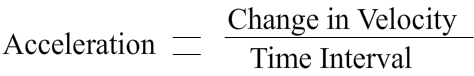OR;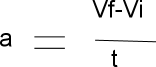In Figure 1.3 given below you see that a car moving in a curved path. While it is traveling its direction is changing with the path. However, we can not observe if there is a change in its speed or not. Can we say that the car has acceleration?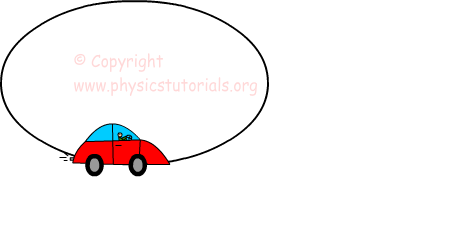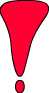The answer of the question is yes. In the definition of acceleration we have said that for having acceleration there must be change in the magnitude or direction of the velocity. In this example we see that the direction of the car also velocity is changing with time. Thus, of course we can say that this car is accelerating.

Now better understanding lets solve some problems.

Example A car starts to move and reaches the velocity 80m/s in 10 seconds. Calculate the acceleration of this car?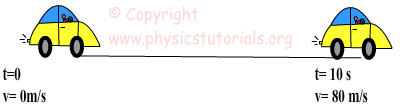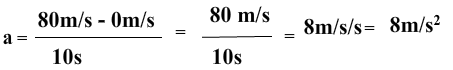Example A boy having 3m/s² acceleration starts to run. Calculate the boy’s final velocity after 15 seconds?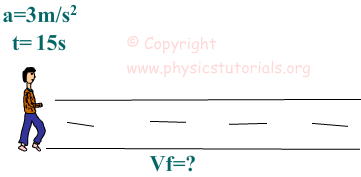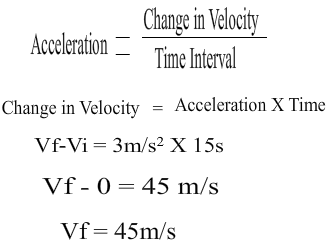Kinematics Exams

Related